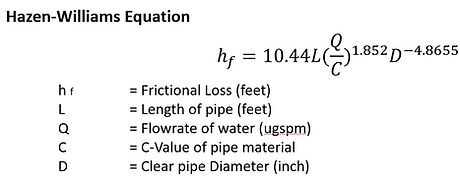top of page

Hydraulic Calculator

A pump’s pressure head can be estimated with many simulation softwares available in the market. Nonetheless, a quick estimation of the overall system’s pressure loss can also provide an idea where the range should be. The pump’s pressure head should be designed to overcome these pressure losses.

The overall system pressure loss arise from losses through the linear length of pipe, losses through fittings or accessories, and losses to overcome gravity (static loss).

Overall System Pressure Loss (feet) = Pipe Loss (A) + Fitting Loss (B) + Static Loss (C)

(A) Pipe Loss

Pressure loss from the linear length of pipe can be represented by the Hazen-Williams Equation, which is explained in more detail in the tab “Linear Length Pipe Loss Calculator”. It is the losses due to the friction against the internal wall of the pipe. Therefore, according to the equation, the friction loss against the wall should be greater if,

1. The Flowrate of water, Q is high

2. The Diameter of the pipe, D is small

3. The Overall Length of Pipe, L is long

4. The C-Value of the pipe is small, i.e., the pipe surface is not smooth(B) Fitting Loss

Different types of fittings result in different amount of losses when water flows pass them. Because the shape of the fitting has more effect on pressure loss it will incur, it is generally accepted that a guide of fitting losses that emphasizes more on the type, rather than the material of the fitting is used.

Most fitting losses can generally be derived from their equivalent length per diameter, Le/D, whose guides from different sources are summarised below.(C) Static Pressure Loss

Static loss is the loss to overcome gravity. For a pump, this is usually the most significant pressure loss to consider. If the pump needs to pump water up for usage above 100 feet height, the static pressure loss is 100 feet.

Example

Determine the pump pressure head required in feet for the system with the following information from designer

1. Flowrate, Q = 30 igpm

2. Type of pipe = Cast iron pipe

3. Total Length of pipe = 60 feet

4. Internal Diameter of Pipe, ID = 2 inch (assuming the same throughout the system)

5. Number of types of fittings throughout the system = 6, i.e.

• 10 numbers of 90  ͦ Elbow

• 2 numbers of T-through branch

• 2 numbers of T-Through run

• 1 number of Alarm Check Valve

• 1 number of Gate Valve

• 1 number of hose coupling

6. Highest level of discharge = 30 feet

7. Required pressure before discharge = 2 bar

Pipe loss

From the C-Value tabulation, Cast Iron has a C-Value of 100Fitting loss

The Equivalent Length per Diameter, Le/D value for different types of fittings can be referred from summarized table above.Static loss

Static pressure loss is encountered if the pump is working to pump water up to its furthest discharge, in which case is 30 feet.You can also download the Excel file with more functions/change of setting in the calculation, including the Tables shown above.